## 22-2 嚙踝蕭嚙踝蕭嚙踝蕭嚙踝蕭嚙踝蕭嚙踝蕭嚙踝蕭嚙踝蕭嚙踝蕭嚙踝蕭嚙踝蕭嚙踝蕭嚙踝蕭嚙?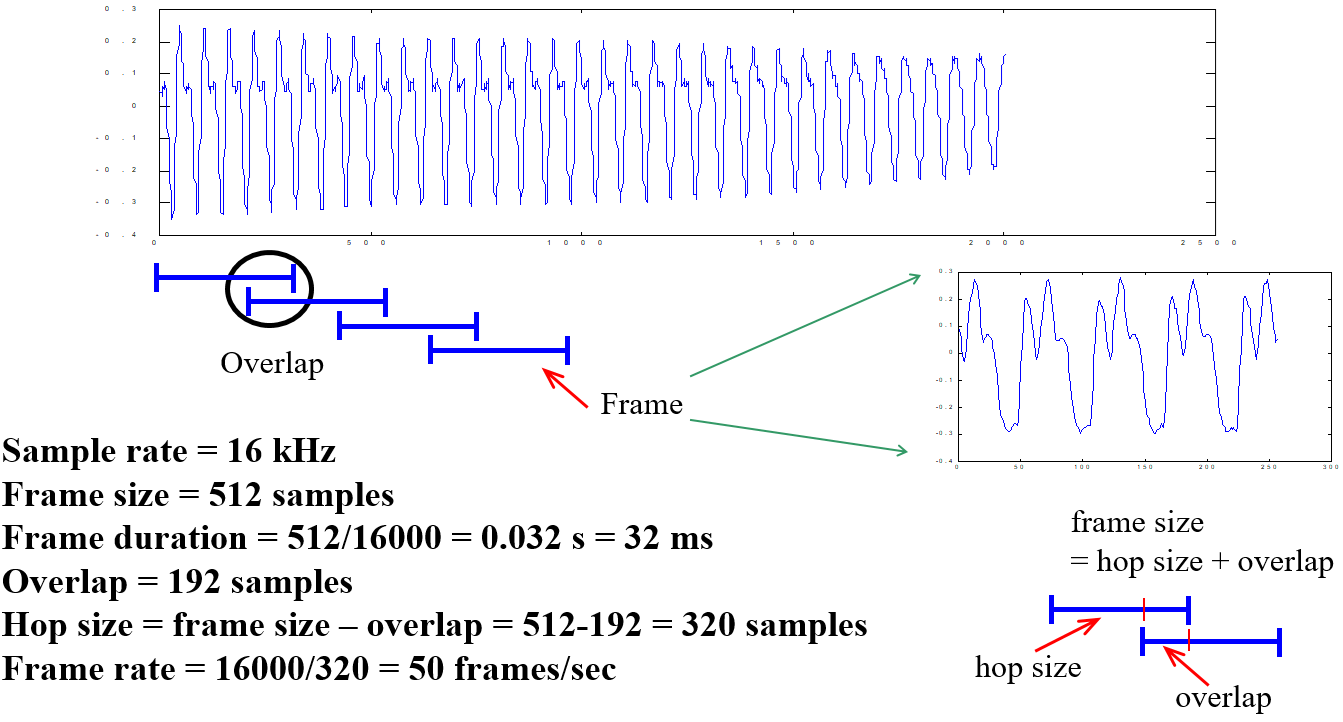圖 5.：由一段音訊中切出音框。

• 音量：代表音訊的強度（intensity）或能量（energy），通常可以使用音訊的震幅來類比，震幅越大，音量越大，音量的單位是分貝（decibel）。
• 音高：代表音訊的高低，例如女生的歌聲會比較高，而男生的歌聲會比較低。通常我們使用在每一秒內出現的基本週期的個數，來代表音高。舉例來說，我們可以先用肉眼觀察音叉的波形，抓出基本週期的位置，然後決定音高，方法如下圖示：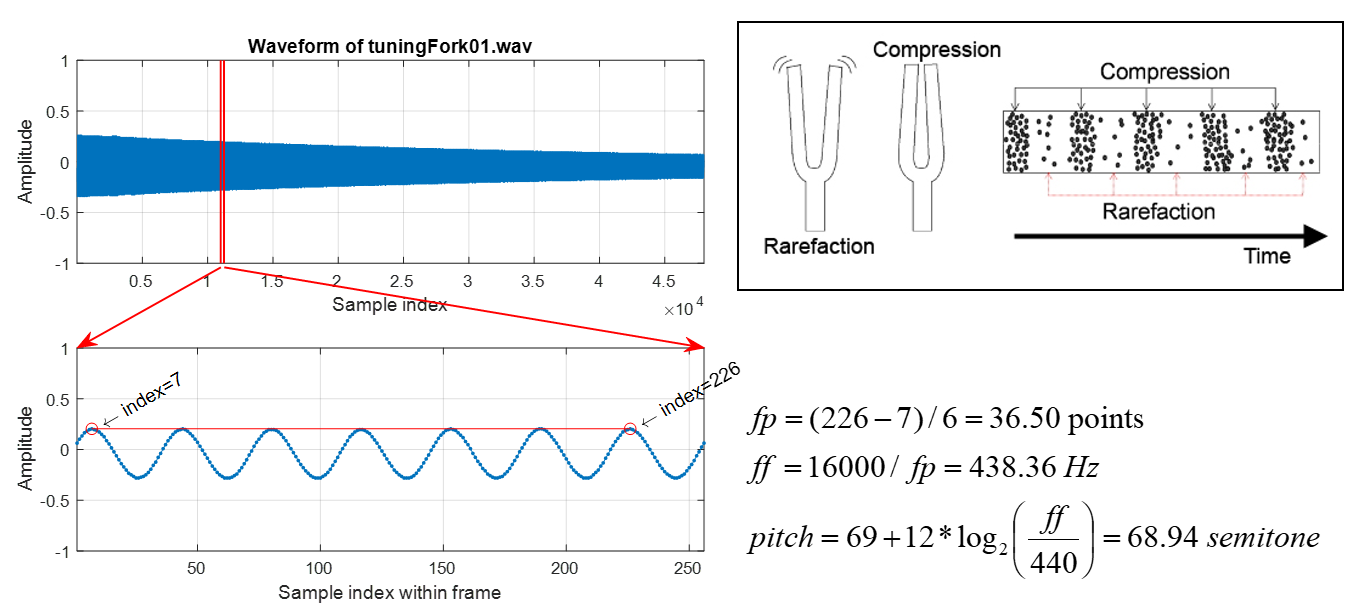圖 5.：由觀察法得到音叉聲音的一個音框的音高。

Example 1: framePitchDisp4tuningFork01.mwaveFile='tuningFork01.wav'; au=myAudioRead(waveFile); y=au.signal; fs=au.fs; index1=11000; frameSize=256; index2=index1+frameSize-1; frame=y(index1:index2); subplot(2,1,1); plot(y); grid on xlabel('Sample index'); ylabel('Amplitude'); title(['Waveform of ', waveFile]); axis([1, length(y), -1 1]); subplot(2,1,2); plot(frame, '.-'); grid on xlabel('Sample index within frame'); ylabel('Amplitude'); point=[7, 226]; % Peaks axis([1, length(frame), -1 1]); periodCount=6; fp=((point(2)-point(1))/periodCount); % fundamental period ff=fs/fp; % fundamental frequency pitch=69+12*log2(ff/440); fprintf('Fundamental period (fp) = (%g-%g)/%g = %g points\n', point(2), point(1), periodCount, fp); fprintf('Fundamental frequency (ff) = %g/%g = %g Hz\n', fs, fp, ff); fprintf('Pitch = %g semitone\n', pitch); % === For plotting arrows, etc % ====== Frame boundary subplot(211); line(index1*[1 1], [-1 1], 'color', 'r', 'linewidth', 1); line(index2*[1 1], [-1 1], 'color', 'r', 'linewidth', 1); % ====== FP coverage subplot(212); line(point, frame(point), 'marker', 'o', 'color', 'red'); % ====== Axis locations subplot(211); loc1=get(gca, 'position'); subplot(212); loc2=get(gca, 'position'); % ====== arrow 1 x1=[loc1(1)+(index1(1)-1)/(length(y)-1)*loc1(3), loc2(1)]; y1=[loc1(2), loc2(2)+loc2(4)]; ah=annotation('arrow', x1, y1, 'color', 'r', 'linewidth', 1); % ======= arrow 2 x2=[loc1(1)+(index2-1)/(length(y)-1)*loc1(3), loc2(1)+loc2(3)]; y2=[loc1(2), loc2(2)+loc2(4)]; ah=annotation('arrow', x2, y2, 'color', 'r', 'linewidth', 1); % ====== Texts indicating start/end indices h1=text(point(1), frame(point(1)), [' \leftarrow index=', int2str(point(1))], 'rotation', 30); h2=text(point(2), frame(point(2)), [' \leftarrow index=', int2str(point(2))], 'rotation', 30); Fundamental period (fp) = (226-7)/6 = 36.5 points Fundamental frequency (ff) = 16000/36.5 = 438.356 Hz Pitch = 68.9352 semitone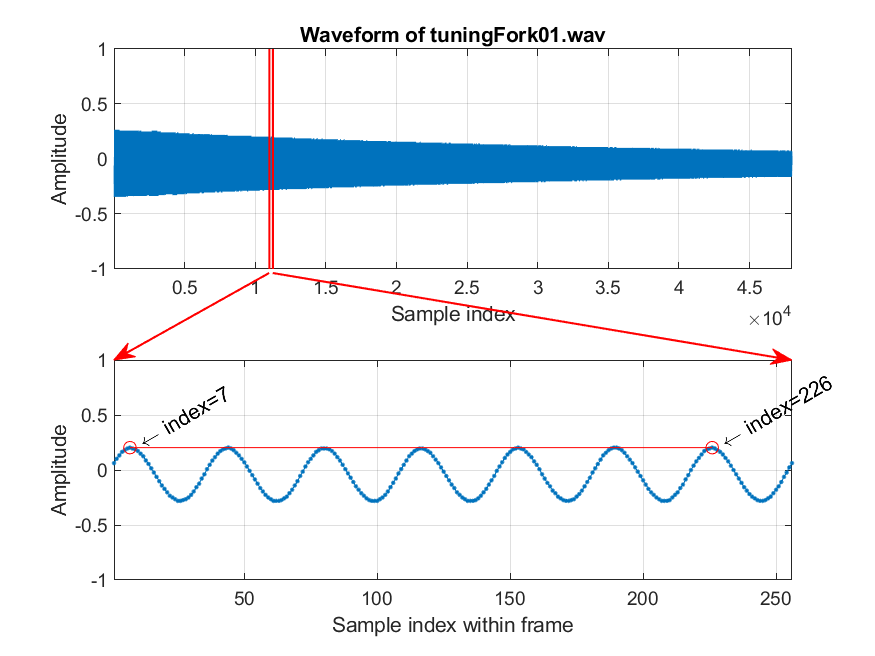在上圖中，我們所處理的聲音內容是音叉的錄音，取樣頻率是 16 KHz（也就是每秒的聲音取樣點是 16000）。我們先切出一個音框，長度是 256 點（時間長度是 512/16000 = 0.032 sec = 32 msec），然後使用觀察法，在這個音框內挑到 6 個完整的基本週期，開始於第 7 點，結束於第 226 點，因此基本週期的時間長度是 (226-7)/6 = 36.5 points，而對應的基本頻率則是 16000/36.5 = 438.36 Hz，代表每秒鐘大約有將近 438 個基本週期。

利用類似的方式，我們也可以決定一個人講話的音高，如下：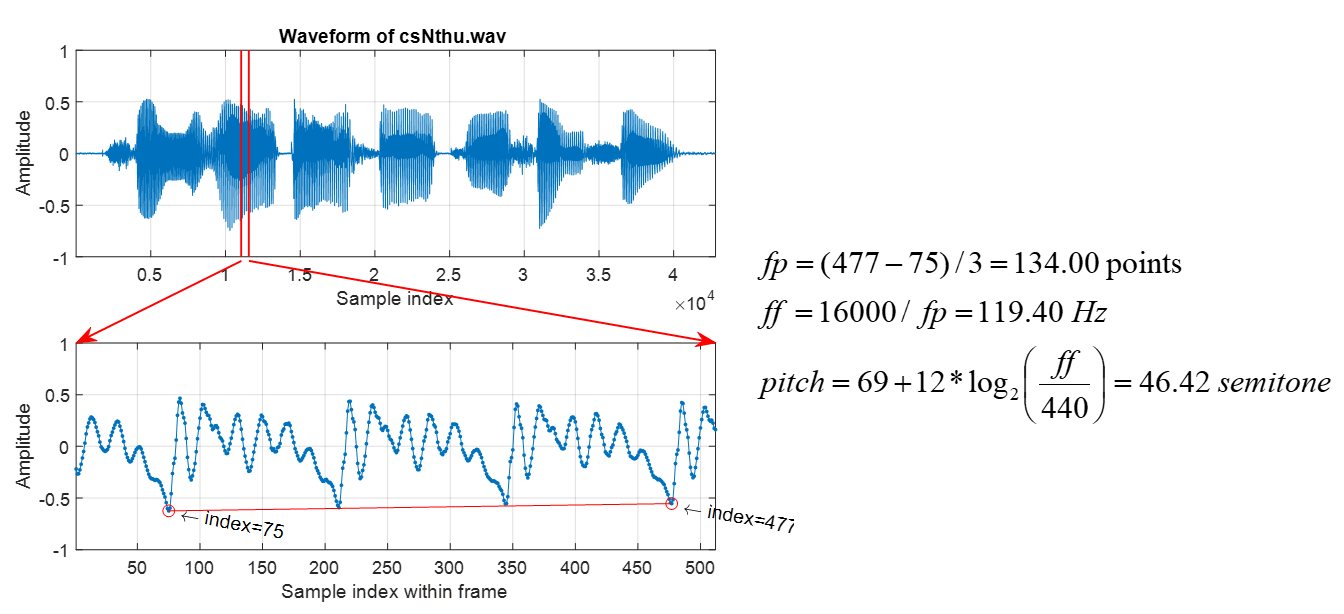圖 5.：由觀察法得到一句語音的一個音框的音高。

Example 2: framePitchDisp4speech01.mwaveFile='csNthu.wav'; au=myAudioRead(waveFile); y=au.signal; fs=au.fs; index1=11050; frameSize=512; index2=index1+frameSize-1; frame=y(index1:index2); subplot(2,1,1); plot(y); grid on xlabel('Sample index'); ylabel('Amplitude'); title(['Waveform of ', waveFile]); axis([1, length(y), -1 1]); subplot(2,1,2); plot(frame, '.-'); grid on xlabel('Sample index within frame'); ylabel('Amplitude'); point=[83, 485]; % Peaks point=[75, 477]; % Valleys axis([1, length(frame), -1 1]); periodCount=3; fp=((point(2)-point(1))/periodCount); % fundamental period ff=fs/fp; % fundamental frequency pitch=69+12*log2(ff/440); fprintf('Fundamental period (fp) = (%g-%g)/%g = %g points\n', point(2), point(1), periodCount, fp); fprintf('Fundamental frequency (ff) = %g/%g = %g Hz\n', fs, fp, ff); fprintf('Pitch = %g semitone\n', pitch); % === For plotting arrows, etc % ====== Frame boundary subplot(211); line(index1*[1 1], [-1 1], 'color', 'r', 'linewidth', 1); line(index2*[1 1], [-1 1], 'color', 'r', 'linewidth', 1); % ====== FP coverage subplot(212); line(point, frame(point), 'marker', 'o', 'color', 'red'); % ====== Axis locations subplot(211); loc1=get(gca, 'position'); subplot(212); loc2=get(gca, 'position'); % ====== arrow 1 x1=[loc1(1)+(index1(1)-1)/(length(y)-1)*loc1(3), loc2(1)]; y1=[loc1(2), loc2(2)+loc2(4)]; ah=annotation('arrow', x1, y1, 'color', 'r', 'linewidth', 1); % ======= arrow 2 x2=[loc1(1)+(index2-1)/(length(y)-1)*loc1(3), loc2(1)+loc2(3)]; y2=[loc1(2), loc2(2)+loc2(4)]; ah=annotation('arrow', x2, y2, 'color', 'r', 'linewidth', 1); % ====== Texts indicating start/end indices h1=text(point(1), frame(point(1)), [' \leftarrow index=', int2str(point(1))], 'rotation', -10); h2=text(point(2), frame(point(2)), [' \leftarrow index=', int2str(point(2))], 'rotation', -10); Fundamental period (fp) = (477-75)/3 = 134 points Fundamental frequency (ff) = 16000/134 = 119.403 Hz Pitch = 46.42 semitone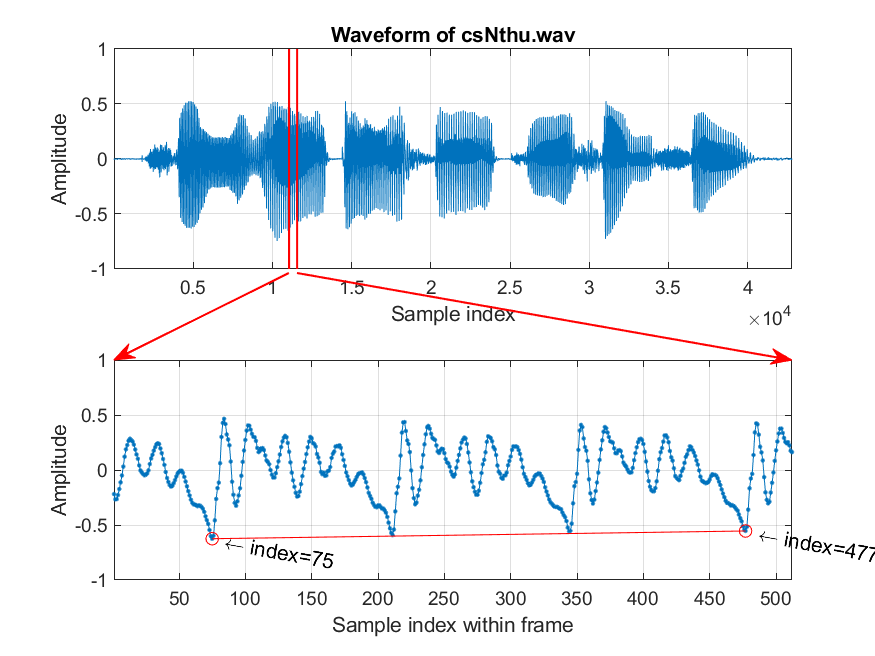在上圖中，我們所處理的聲音內容是「清華大學資訊系」，取樣頻率是 16 KHz（也就是每秒的聲音取樣點是 16000）。我們先切出一個音框，長度是 512 點（時間長度是 512/16000 = 0.032 sec = 32 msec），然後使用觀察法，在這個音框內挑到 3 個完整的基本週期，開始於第 75 點，結束於第 477 點，因此基本週期的時間長度是 (477-75)/3 = 134 points，而對應的基本頻率則是 16000/134 = 119.40 Hz，代表每秒鐘大約有將近 119 個基本週期。

Hint
在上述觀察法中，基本週期的偵測是靠肉眼來達成，而決定每一個基本週期開始的點，通常稱為音高週期起點（pitch mark），可以是波峰（如前述的音叉範例）或波谷（如前述的人聲範例）。

由於我們人耳對於聲音高低的感覺，並不是直接和聲音的基本頻率成正比，而是和聲音的基本頻律的對數值成正比，因此我們可以使用半音差（semitone）來表示音高，公式如下： $$pitch = 69 + 12 \log_2 \left(\frac{freq}{440} \right)$$ 其中 $freq$ 是以 Hz 為單位的基本頻率值，而 $pitch$ 則是以 semitone 為單位的音高值，這個音高值又稱為 MIDI number，可以直接對應到鋼琴的每一個琴鍵，例如當 $freq=440$ 時，所對應到的音高是 $pitch=69$，這就是鋼琴的中央 La（或稱 middle A、A440、A4）鍵。

• 音色：代表音訊的內容，例如「ㄚ」和「ㄛ」的發音方式不同，就會產生不同的音色，另外不同樂器所產生的聲音，也是屬於不同的音色。通常我們使用音訊在不同頻率的能量分布，來代表音色，因此經由快速傅立葉轉換（fast Fourier transform, FFT）來將一個音框的訊號轉成幅度頻譜（magnitude spectrum），就可以做為音色的特徵。但以幅度頻譜的特徵，常受到音高的影響，而有協波（harmonics）的現象，因此另一個典型的音色特徵是 MFCC (mel-frequency cepstral coefficients) ，比較不會受到協波的影響，可以代表人耳對音色的感受，此特徵常用在語音辨識。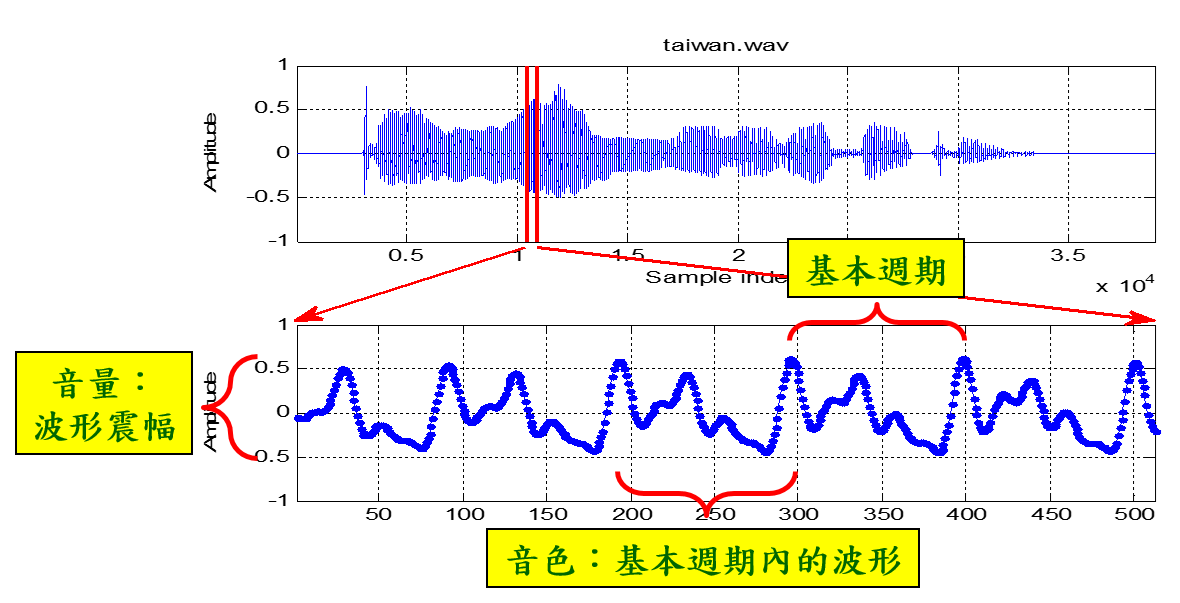圖 5.：聲學特徵顯示於時域的對應表現。

Example 3: frameDisp4fea01.mauFile='taiwanUniversity.wav'; au=myAudioRead(auFile); n=2; au.fs=au.fs/n; au.signal=au.signal(1:n:end, 1); % Down sampling index1=round(0.66*au.fs); frameSize=256; index2=index1+frameSize-1; frame=au.signal(index1:index2); % Take the frame for display audiowrite('frame.wav', frame, au.fs); subplot(2,1,1); plot(au.signal); grid on xlabel('Sample index'); ylabel('Amplitude'); title(auFile); %xlabel('取樣點索引值'); ylabel('波形振幅'); title(auFile); axis([1, length(au.signal), -1 1]); line(index1*[1 1], [-1 1], 'color', 'm', 'linewidth', 1); line(index2*[1 1], [-1 1], 'color', 'm', 'linewidth', 1); subplot(2,1,2); plot(frame, '.-'); grid on xlabel('Sample index within the frame'); ylabel('Amplitude'); %xlabel('音框內的取樣點索引值'); ylabel('波形振幅'); axis([1, length(frame), -1 1]); %boxOverlay([60.5 -0.7 52 1.4], 'r', 1, '音色：基本週期內的波形', 'top'); message=sprintf('Timbre:\nWaveform in a fundamental period'); boxOverlay([60.5 -0.5 52 1.2], 'r', 1, message, 'top'); subplot(211); loc1=get(gca, 'position'); subplot(212); loc2=get(gca, 'position'); %% ===== arrow 1 for closeup x1=[loc1(1)+(index1(1)-1)/(length(au.signal)-1)*loc1(3), loc2(1)]; y1=[loc1(2), loc2(2)+loc2(4)]; ah=annotation('arrow', x1, y1, 'color', 'm', 'linewidth', 1); %% ====== arrow 2 for closeup x2=[loc1(1)+(index2-1)/(length(au.signal)-1)*loc1(3), loc2(1)+loc2(3)]; y2=[loc1(2), loc2(2)+loc2(4)]; ah=annotation('arrow', x2, y2, 'color', 'm', 'linewidth', 1); %% Double arrow for fundamental period axisLimit=axis; % axisLimit=[1, 256, -1, 1] xPos=[171, 223]; yPos=[0.65, 0.65]; xRel=loc2(1)+(xPos-axisLimit(1))/(axisLimit(2)-axisLimit(1))*loc2(3); yRel=loc2(2)+(yPos-axisLimit(3))/(axisLimit(4)-axisLimit(3))*loc2(4); ah=annotation('doublearrow', xRel, yRel, 'color', 'r'); textH=text(mean(xPos), mean(yPos), 'Fundamental period', 'horizontal', 'center', 'vertical', 'bottom'); %% Double arrow for volume axisLimit=axis; % axisLimit=[1, 256, -1, 1] xPos=axisLimit(2)+[1, 1]; yPos=[min(frame), max(frame)]; xRel=loc2(1)+(xPos-axisLimit(1))/(axisLimit(2)-axisLimit(1))*loc2(3); yRel=loc2(2)+(yPos-axisLimit(3))/(axisLimit(4)-axisLimit(3))*loc2(4); ah=annotation('doublearrow', xRel, yRel, 'color', 'r'); textH=text(mean(xPos), mean(yPos), 'Volume', 'horizontal', 'center', 'vertical', 'top', 'rotation', 90);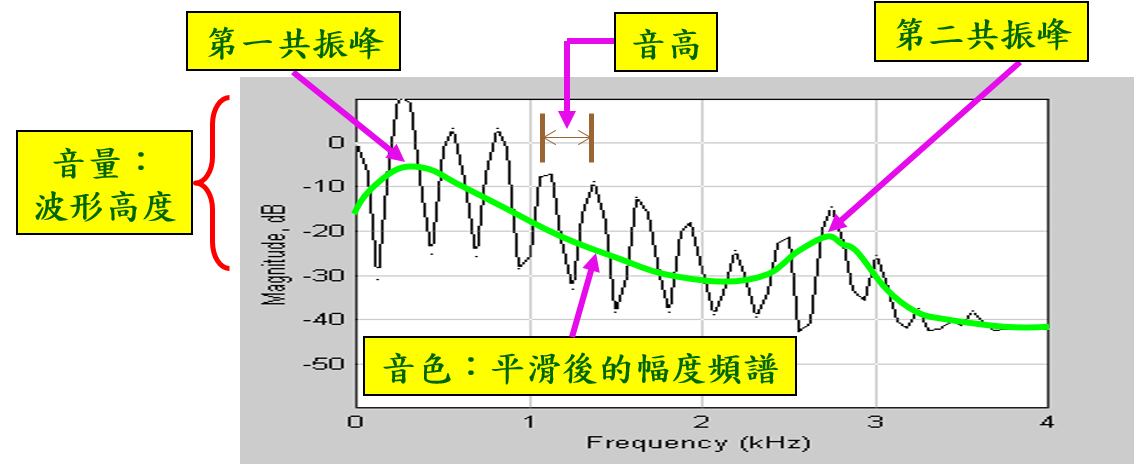圖 5.：聲學特徵顯示於頻域的對應表現。

Example 4: frameDisp4fea02.mwaveFile='taiwanUniversity.wav'; au=myAudioRead(waveFile); n=2; au.fs=au.fs/n; au.signal=au.signal(1:n:end, 1); % Down sampling index1=round(0.66*au.fs); frameSize=256; index2=index1+frameSize-1; frame=au.signal(index1:index2); % Take the frame for display frameBasic=frame.*hanning(frameSize); frameExtended=zeros(8*frameSize, 1); frameExtended(1:frameSize)=frameBasic; [magSpec, phaseSpec, freqVec, powerSpecInDb]=fftOneSide(frameExtended, au.fs); plot(freqVec, powerSpecInDb); grid on title('Power spectrum'); xlabel('Frequency (Hz)'); ylabel('Power (dB)'); axis tight %xlabel('頻率 (Hz)'); ylabel('振幅 (dB)'); order=10; freqNormalized=freqVec/max(freqVec); p=polyfit(freqNormalized, powerSpecInDb, order); f=polyval(p, freqNormalized); line(freqVec, f, 'color', 'g', 'linewidth', 2); %% Plot FF axisLimit=axis; axisLoc=get(gca, 'position'); xPos=[945.3125, 1085.9375]; yPos=[6.9, 6.9]; xRel=axisLoc(1)+((xPos-axisLimit(1))/(axisLimit(2)-axisLimit(1)))*axisLoc(3); yRel=axisLoc(2)+((yPos-axisLimit(3))/(axisLimit(4)-axisLimit(3)))*axisLoc(4); ah=annotation('doublearrow', xRel, yRel, 'color', 'r'); textH=text(mean(xPos), mean(yPos), 'FF', 'horizontal', 'center', 'vertical', 'bottom'); %% Plot first formant [maxValue, maxId]=max(f); xPos=[freqVec(maxId)+100, freqVec(maxId)]; yPos=[maxValue+20, maxValue]; line(xPos(2), yPos(2), 'marker', 'o', 'color', 'r'); xRel=axisLoc(1)+((xPos-axisLimit(1))/(axisLimit(2)-axisLimit(1)))*axisLoc(3); yRel=axisLoc(2)+((yPos-axisLimit(3))/(axisLimit(4)-axisLimit(3)))*axisLoc(4); ah=annotation('arrow', xRel, yRel, 'color', 'r'); textH=text(xPos(1), yPos(1), 'First formant', 'horizontal', 'center', 'vertical', 'bottom'); %% Plot second formant f(1:round(length(f)/2))=-inf; [maxValue, maxId]=max(f); xPos=[freqVec(maxId)+100, freqVec(maxId)]; yPos=[maxValue+30, maxValue]; line(xPos(2), yPos(2), 'marker', 'o', 'color', 'r'); xRel=axisLoc(1)+((xPos-axisLimit(1))/(axisLimit(2)-axisLimit(1)))*axisLoc(3); yRel=axisLoc(2)+((yPos-axisLimit(3))/(axisLimit(4)-axisLimit(3)))*axisLoc(4); ah=annotation('arrow', xRel, yRel, 'color', 'r'); textH=text(xPos(1), yPos(1), 'Second formant', 'horizontal', 'center', 'vertical', 'bottom'); %% Plot timbre curve point=[1184, -41.83]; xPos=[point(1)+300, point(1)]; yPos=[point(2)+25, point(2)]; xRel=axisLoc(1)+((xPos-axisLimit(1))/(axisLimit(2)-axisLimit(1)))*axisLoc(3); yRel=axisLoc(2)+((yPos-axisLimit(3))/(axisLimit(4)-axisLimit(3)))*axisLoc(4); ah=annotation('arrow', xRel, yRel, 'color', 'r'); textH=text(xPos(1), yPos(1), 'Timbre: Smoothed power spectrum', 'horizontal', 'center', 'vertical', 'bottom'); %% Plot energy point=[freqVec(1), powerSpecInDb(1)]; line(point(1), point(2), 'marker', 'o', 'color', 'r'); xPos=[point(1)+300, point(1)]; yPos=[point(2)-25, point(2)]; xRel=axisLoc(1)+((xPos-axisLimit(1))/(axisLimit(2)-axisLimit(1)))*axisLoc(3); yRel=axisLoc(2)+((yPos-axisLimit(3))/(axisLimit(4)-axisLimit(3)))*axisLoc(4); ah=annotation('arrow', xRel, yRel, 'color', 'r'); textH=text(xPos(1), yPos(1), 'Energy (volume)', 'horizontal', 'center', 'vertical', 'top');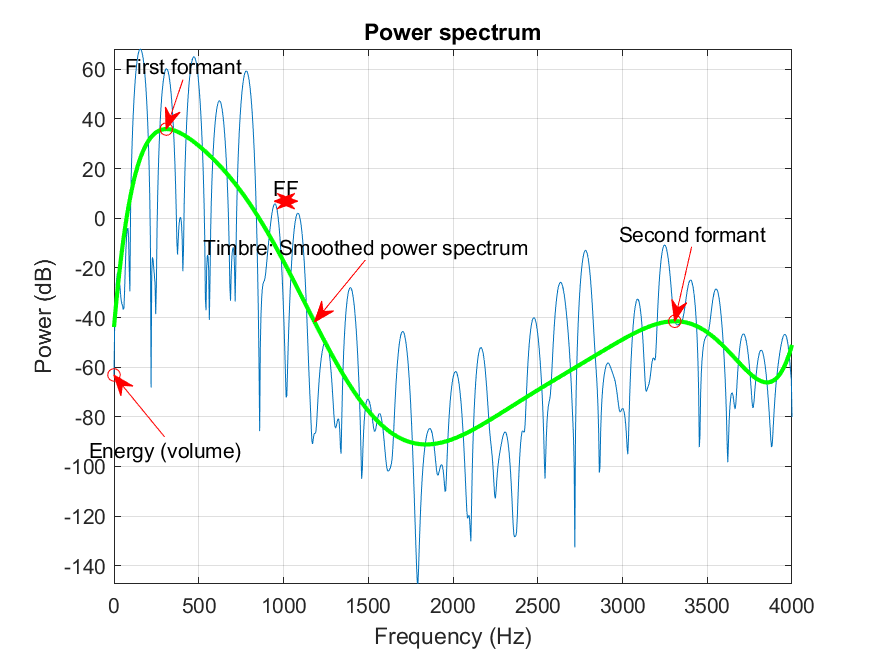#### 作業

1. 一段音訊的取樣頻率是 16 kHz，若是音框長度是 320 個取樣點，請回答下列問題：
1. 如果音框之間的重疊是 120 點，那麼對應的音框率是？
2. 如果音框率是 100 frame/sec，則音框之間的重疊應該是幾點？
2. 假設我從我的語音訊號抽出一個音框，如下圖。如果取樣頻率是 8 kHz，請計算這個音框的基本頻率。（在選取基本週期來進行平均時，基本週期的個數必須越多越好，以求穩定。）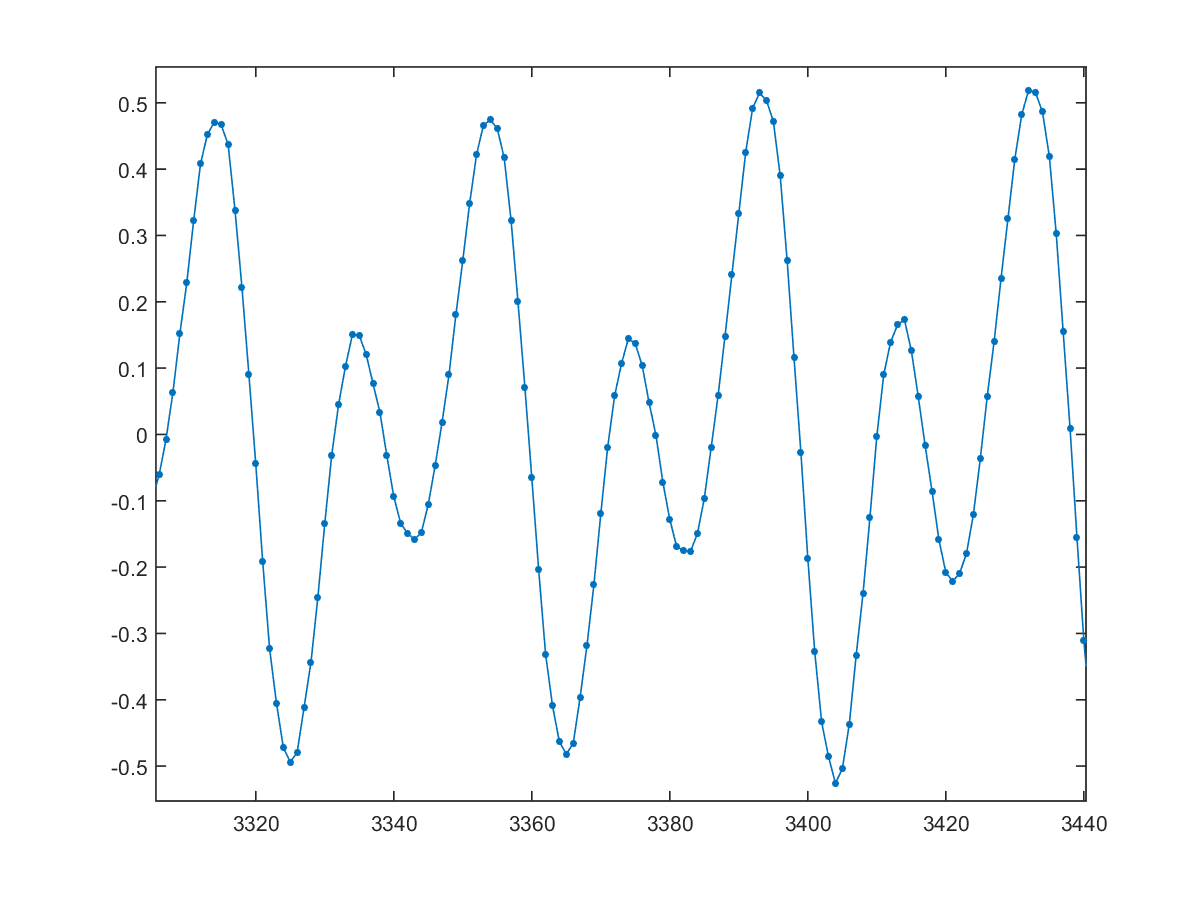圖 5.：請由此音框計算對應的基本頻率。

3. 請從網路上尋找資訊，來解釋下列名詞（請盡量使用數學方程式來說明），並說明在日常生活中，何時會遇到這些現象：
1. 拍頻（beat frequency）
2. 杜普勒效應（Doppler effect）

Audio Signal Processing and Recognition (音訊處理與辨識)